##### Quantum Physics For DummiesIn quantum physics, you can find the wave function of the ground state of a quantum oscillator, such as the one shown in the figure, which takes the shape of a gaussian curve.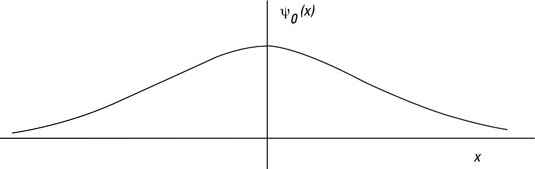The ground state of a quantum mechanical harmonic oscillator.

As a gaussian curve, the ground state of a quantum oscillator is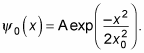How can you figure out A? Wave functions must be normalized, so the following has to be true: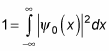Substituting for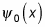gives you this next equation: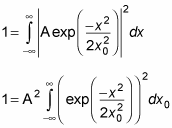You can evaluate this integral to be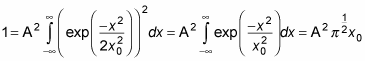Therefore,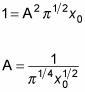This means that the wave function for the ground state of a quantum mechanical harmonic oscillator is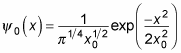Cool. Now you’ve got an exact wave function.